﻿ 分子机器中链状分子热传导性质的理论研究 Theoretical Study on the Heat Conductivities of Chain Molecules in Molecular Machines

Modern Physics
Vol.07 No.06(2017), Article ID:22637,8 pages
10.12677/MP.2017.76026

Theoretical Study on the Heat Conductivities of Chain Molecules in Molecular Machines

Jianping Huang1*, Jing Tang2

1College of Information Science and Technology, Hunan Normal University, Changsha Hunan

2College of Physics and Information Science, Hunan Normal University, Changsha Hunan

*通讯作者。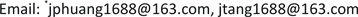Received: Oct. 20th, 2017; accepted: Nov. 4th, 2017; published: Nov. 10th, 2017ABSTRACT

The formula for energy flux of chain molecule vibrations in molecular machines was derived based on the lattice dynamics, and then the formula for heat conductivities of chain molecules was derived based on energy flux formula and Green-Kubo formula. In view of the fact that the linewidths of all phonons should be needed during the calculations of the heat conductivities of chain molecules, the formula for phonons’ linewidths was derived based on lattice dynamics and Green’s function theory. Finally the numerical calculations were carried out, and the results show that the heat conductivities of chain molecules increase and tend to infinity when their lengths increase and tend to infinity, and there is a big difference between the heat conductivities of a chain molecule and a cyclic molecule with the same length. The numerical results also show that the main contributions to the heat conductivities of long chain molecules were made by phonons with short wave vectors.

Keywords:Chain Molecule, Heat Conductivity, Energy Flux, Linewidth of Phonon, Lattice Dynamics

1湖南师范大学信息科学与工程学院，湖南 长沙

2湖南师范大学物理与信息科学学院，湖南 长沙1. 引言

2. 链状分子的晶格动力学与热传导理论

${u}_{l}=\sum _{j=1}^{N-1}\sqrt{\frac{\hslash }{Nm{\omega }_{j}}}{A}_{j}\mathrm{cos}\left(l-\frac{1}{2}\right)\frac{j\text{π}}{N}$ (1)

${p}_{l}=-i\sum _{j=1}^{N-1}\sqrt{\frac{\hslash m{\omega }_{j}}{N}}{B}_{j}\mathrm{cos}\left(l-\frac{1}{2}\right)\frac{j\text{π}}{N}$ (2)

${\omega }_{j}=2\sqrt{\frac{\beta }{m}}\mathrm{sin}\frac{j\text{π}}{2N}$ (3)

$S=\frac{a\beta }{2m}\sum _{l=1}^{N}\left({u}_{l}-{u}_{l-1}\right)\left({p}_{l}+{p}_{l-1}\right)$ (4)

$S=\sum _{j=1}^{N-1}\sum _{{j}^{\prime }=1}^{N-1}{A}_{j}{B}_{{j}^{\prime }}\eta \left(j,{j}^{\prime }\right)$ (5)

$\eta \left(j,{j}^{\prime }\right)=\frac{i2\hslash {V}_{j}{V}_{{j}^{\prime }}\sqrt{{\omega }_{j}{\omega }_{{j}^{\prime }}}}{Na}\frac{{\omega }_{{j}^{\prime }}}{{\omega }_{{j}^{\prime }}{}^{2}-{\omega }_{j}{}^{2}}$ (6)

${V}_{j}=a\sqrt{\frac{\beta }{m}}\mathrm{cos}\frac{\text{π}j}{2N}$ (7)

$\lambda =\frac{1}{2Na{k}_{B}{T}^{2}}{\int }_{-\infty }^{+\infty }\text{d}t〈S\left(0\right)S\left(t\right)〉$ (8)

$〈abcd〉=〈ab〉〈cd〉+〈ac〉〈bd〉+〈ad〉〈bc〉$ (9)

$\begin{array}{l}\lambda =\frac{-1}{4Na{k}_{B}{T}^{2}}\sum _{j=1}^{N-1}\sum _{j\text{'}=1}^{N-1}{\int }_{-\infty }^{+\infty }\text{d}t\left\{{\left[\eta \left(j,{j}^{\prime }\right)+\eta \left({j}^{\prime },j\right)\right]}^{2}\left[〈{a}_{j}\left(0\right){a}_{j}^{+}\left(t\right)〉〈{a}_{{j}^{\prime }}\left(0\right){a}_{{j}^{\prime }}^{+}\left(t\right)〉\end{array}$ $+〈{a}_{j}^{+}\left(0\right){a}_{j}\left(t\right)〉〈{a}_{{j}^{\prime }}^{+}\left(0\right){a}_{{j}^{\prime }}\left(t\right)〉\right]\text{\hspace{0.17em}}\text{\hspace{0.17em}}\text{\hspace{0.17em}}\text{\hspace{0.17em}}+{\left[\eta \left(j,{j}^{\prime }\right)-\eta \left({j}^{\prime },j\right)\right]}^{2}\left[〈{a}_{j}\left(0\right){a}_{j}^{+}\left(t\right)〉〈{a}_{{j}^{\prime }}^{+}\left(0\right){a}_{{j}^{\prime }}\left(t\right)〉$ $+〈{a}_{j}^{+}\left(0\right){a}_{j}\left(t\right)〉〈{a}_{{j}^{\prime }}\left(0\right){a}_{{j}^{\prime }}^{+}\left(t\right)〉\right]\right\}$ (10)

$〈{a}_{j}\left(0\right){a}_{j}^{+}\left(t\right)〉=\left({\overline{n}}_{j}+1\right){\text{e}}^{i{\omega }_{j}t-{\Gamma }_{j}|t|}\text{}$ (11)

$〈{a}_{j}^{+}\left(0\right){a}_{j}\left(t\right)〉={\overline{n}}_{j}{\text{e}}^{-i{\omega }_{j}t-{\Gamma }_{j}|t|}$ (12)

${\overline{n}}_{j}=\frac{1}{{\text{e}}^{\hslash {\omega }_{j}/{k}_{B}T}-1}$ (13)

$\lambda =\frac{4{\hslash }^{2}}{{k}_{B}{T}^{2}{N}^{3}{a}^{3}}\sum _{j=1}^{N-1}\sum _{j\text{'}=1}^{N-1}\sum _{±}\left[\left({\overline{n}}_{j}+\frac{1}{2}\right)\left({\overline{n}}_{{j}^{\prime }}+\frac{1}{2}\right)±\frac{1}{4}\right]\frac{{\omega }_{j}{\omega }_{{j}^{\prime }}{V}_{j}^{2}{V}_{{j}^{\prime }}^{2}}{{\left({\omega }_{j}±{\omega }_{{j}^{\prime }}\right)}^{2}}\frac{\left({\Gamma }_{j}+{\Gamma }_{{j}^{\prime }}\right)}{{\left({\omega }_{j}±{\omega }_{{j}^{\prime }}\right)}^{2}+{\left({\Gamma }_{j}+{\Gamma }_{{j}^{\prime }}\right)}^{2}}$ (14)

3. 链状分子的声子格林函数与声子谱线宽度

$H=\sum _{j}\left({a}_{j}^{+}{a}_{j}+\frac{1}{2}\right)\hslash {\omega }_{j}+\sum _{{j}_{1}{j}_{2}j}{V}_{3}\left({j}_{1},{j}_{2},j\right){A}_{{j}_{1}}{A}_{{j}_{2}}{A}_{j}$ (15)

${|{V}_{3}\left({j}_{1},{j}_{2},j\right)|}^{2}=\frac{4{\gamma }^{2}{\hslash }^{3}{m}^{2}{\left({\omega }_{{j}_{1}}{\omega }_{{j}_{2}}{\omega }_{j}\right)}^{3}{\left({V}_{j}{V}_{{j}_{1}}{V}_{{j}_{2}}\right)}^{2}}{9{N}^{3}{\beta }^{5}{a}^{6}{\left({\omega }_{j+{j}_{1}+{j}_{2}}{\omega }_{j+{j}_{1}-{j}_{2}}{\omega }_{j-{j}_{1}+{j}_{2}}{\omega }_{j-{j}_{1}-{j}_{2}}\right)}^{2}}$ (16)

${G}_{j,j}\left(t;{t}^{\prime }\right)=-i\Theta \left(t-{t}^{\prime }\right)〈\left[{a}_{j}^{+}\left(t\right),{a}_{j}\left({t}^{\prime }\right)\right]〉$ (17)

${G}_{j,j}\left(\omega \right)=\frac{1}{2\text{π}\left[\omega -{\omega }_{j}-{M}_{j}\left(\omega \right)\right]}$ (18)

${M}_{j}\left(\omega \right)=\frac{36}{{\hslash }^{2}}\sum _{{j}_{1}=1}^{N-1}\sum _{{j}_{2}=1}^{N-1}\sum _{±}{|{V}_{3}\left({j}_{1},{j}_{2},j\right)|}^{2}\left[\left({\overline{n}}_{{j}_{2}}+\frac{1}{2}\right)±\left({\overline{n}}_{{j}_{1}}+\frac{1}{2}\right)\right]\frac{{\omega }_{{j}_{1}}±{\omega }_{{j}_{2}}}{{\left[\omega +i\left({\Gamma }_{{j}_{1}}+{\Gamma }_{{j}_{2}}\right)\right]}^{2}-{\left({\omega }_{{j}_{2}}±{\omega }_{{j}_{1}}\right)}^{2}}$ (19)

${M}_{j}\left(\omega \right)$ 中ω取值 ${\omega }_{j}$ ，并分解为实部 ${\Delta }_{j}$ 和虚部 $-i{\Gamma }_{j}$ 之和。由于格林函数的极点实部代表声子频谱，而极点虚部代表声子谱线宽度，故得以下声子谱线宽度公式：

${\Gamma }_{j}=\frac{72}{{\hslash }^{2}}\sum _{{j}_{1}=1}^{N-1}\sum _{{j}_{2}=1}^{N-1}\sum _{±}{|{V}_{3}\left({j}_{1},{j}_{2},j\right)|}^{2}\left[\left({n}_{{j}_{2}}+\frac{1}{2}\right)±\left({n}_{{j}_{1}}+\frac{1}{2}\right)\right]\frac{{\omega }_{j}\left({\Gamma }_{{j}_{1}}+{\Gamma }_{{j}_{2}}\right)\left({\omega }_{{j}_{1}}±{\omega }_{{j}_{2}}\right)}{{\left[{\omega }_{j}^{2}-{\left({\omega }_{{j}_{2}}±{\omega }_{{j}_{1}}\right)}^{2}\right]}^{2}+4{\omega }_{j}^{2}{\left({\Gamma }_{{j}_{1}}+{\Gamma }_{{j}_{2}}\right)}^{2}}$ (20)

${\Gamma }_{{k}_{1}}+{\Gamma }_{{k}_{2}}$ 取值为 ${0}^{+}$ 时，(20)式转化为：

${\Gamma }_{k}=\frac{18\text{π}}{{\hslash }^{2}}\sum _{{j}_{1}=1}^{N-1}\sum _{{j}_{2}=1}^{N-1}\sum _{±}{|{V}_{3}\left({j}_{1},{j}_{2},j\right)|}^{2}\left[\left({\overline{n}}_{{j}_{2}}+\frac{1}{2}\right)$ $±\left({\overline{n}}_{{j}_{1}}+\frac{1}{2}\right)\right]\left\{\delta \left[{\omega }_{j}-\left({\omega }_{{j}_{1}}±{\omega }_{{j}_{2}}\right)\right]-\delta \left[{\omega }_{j}+\left({\omega }_{{j}_{1}}±{\omega }_{{j}_{2}}\right)\right]\right\}$ (21)

${\Gamma }_{j}=\frac{72}{{\hslash }^{2}}\sum _{{j}_{1}=1}^{N-1}\sum _{{j}_{2}=1}^{N-1}\sum _{±}{|{V}_{3}\left({j}_{1},{j}_{2},j\right)|}^{2}\left[\left({n}_{{j}_{2}}+\frac{1}{2}\right)±\left({n}_{{j}_{1}}+\frac{1}{2}\right)\right]\frac{{\omega }_{j}\left({\Gamma }_{j}+{\Gamma }_{{j}_{1}}+{\Gamma }_{{j}_{2}}\right)\left({\omega }_{{j}_{1}}±{\omega }_{{j}_{2}}\right)}{{\left[{\omega }_{j}^{2}-{\left({\omega }_{{j}_{2}}±{\omega }_{{j}_{1}}\right)}^{2}\right]}^{2}+4{\omega }_{j}^{2}{\left({\Gamma }_{j}+{\Gamma }_{{j}_{1}}+{\Gamma }_{{j}_{2}}\right)}^{2}}$ (22)

4. 数值计算结果与分析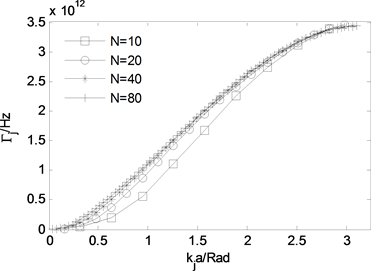Figure 1. Phonon linewidth vs. wave vector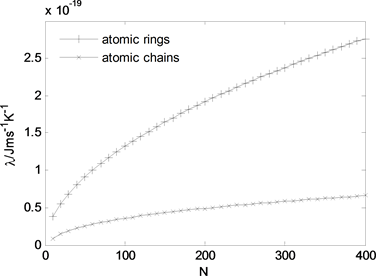Figure 2. Heat conductivity of chain molecule vs. its length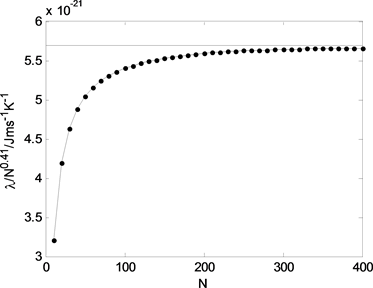Figure 3. Heat conductivity of chain molecule vs. its length (ordinate: $\lambda /{N}^{0.41}$ )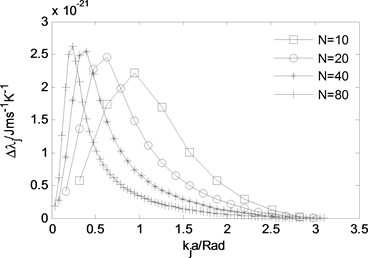Figure 4. The contribution made by phonon to heat conductivity vs. wave vector

5. 结论

Theoretical Study on the Heat Conductivities of Chain Molecules in Molecular Machines[J]. 现代物理, 2017, 07(06): 227-234. http://dx.doi.org/10.12677/MP.2017.76026

1. 1. Cheng, C., Stoddart, J.F. (2016) Wholly Synthetic Molecular Machine. Materials Chemistry and Physics, 17, 1780- 1793. https://doi.org/10.1002/cphc.201501155

2. 2. Ruangsupapichat, N., Pollard, M.M., Harutyunyan, S.R., et al. (2011) Reversing the Direction in a Light-Driven Rotary Molecular Motor. Nature Chemistry, 3, 53-60. https://doi.org/10.1038/nchem.872

3. 3. Kudernac, T., Ruangsupapichat, N., Parschau, M., et al. (2011) Electrically Driven Directional Motion of a Four-Wheeled Molecule on a Metal Surface. Nature, 479, 208-211. https://doi.org/10.1038/nature10587

4. 4. Badjic, J.D., Balzani, V., Credi, A., et al. (2004) A Molecular Elevator. Science, 303, 1845-1849. https://doi.org/10.1126/science.1094791

5. 5. Liu, Y., Flood, A.H., Bonvallet, P.A., et al. (2005) Linear Artificial Molecular Muscles. Journal of the American Chemical Society, 127, 9745-9759. https://doi.org/10.1021/ja051088p

6. 6. Koumura, N., Zijlstra, R.W., Van Delden, R.A., et al. (1999) Light-Driven Monodirectional Molecular Rotor. Nature, 401, 152-155.

7. 7. 黄建平, 唐婧. 分子机器中环状分子热传导性质的理论研究[J]. 现代物理, 2017, 7(5): 169-174.

8. 8. 唐婧, 黄建平. 分子机器中链状分子热膨胀性质的理论研究[J]. 现代物理,2017, 7(6): 221-226

9. 9. Frizzera, W., Viliani, G., Montagna, M., et al. (1997) One-Dimensional Dynamics of Lattice Thermal Transport. Journal of Physics: Condensed Matter, 9, 10867-10879.

10. 10. Semwal, B.S. and Sharma, P.K. (1972) Heat Conductivity of an Anharmonic Crystal. Physical Review B, 5, 3909-3913. https://doi.org/10.1103/PhysRevB.5.3909

11. 11. Maradudin, A.A. and Fein, A.E. (1973) Scattering of Neutrons by an Anharmonic Crystal. Physical Review B, 7, 2589-2608.

12. 12. Turney, J.E., Landry, E.S., Mcgaughey, A.J.H., et al. (2009) Predicting Phonon Properties and Heat Conductivity from Anharmonic Lattice Dynamics Calculations and Molecular Dynamics Simulations. Physical Review B, 79, Article ID: 064301.

13. 13. 鲍华. 固体氩的晶格热导率的非简谐晶格动力学计算[J]. 物理学报, 2013, 62(18): 1-7.

14. 14. Hyzorek, K. and Tretiakov, K.V. (2016) Thermal Conductivity of Liquid Argon in Nanochannels from Molecular Dynamics Simulations. The Journal of Chemical Physics, 144, Article ID: 194507. https://doi.org/10.1063/1.4949270

15. 15. Zhao, Y., Xue, B. X., Wang, Y.M., et al. (2009) Thermal Transport in One-Dimensional FPU-FK Lattices. Communications in Theoretical Physics, 52, 507-510.

16. 16. Dhar, A. (2008) Heat Transport in Low-Dimensional Systems. Advances in Physics, 57, 457-537.